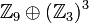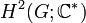# Group cohomology of groups of order 27

(diff) ← Older revision | Latest revision (diff) | Newer revision → (diff)
This article gives specific information, namely, group cohomology, about a family of groups, namely: groups of order 27.
View group cohomology of group families | View group cohomology of groups of a particular order |View other specific information about groups of order 27
Group GAP ID 2nd part Nilpotency class Cohomology information page
cyclic group:Z27 1 1 See group cohomology of finite cyclic groups
direct product of Z9 and Z3 2 1 See group cohomology of direct product of cyclic group of prime-square order and cyclic group of prime order
prime-cube order group:U(3,3) 3 2 group cohomology of prime-cube order group:U(3,3)
M27 4 2 group cohomology of M27
elementary abelian group:E27 5 1 See group cohomology of elementary abelian group of prime-cube order

With the exception of the zeroth homology group and cohomology group, all homology and cohomology groups over all possible abelian groups are 3-groups.

## Homology groups for trivial group action

FACTS TO CHECK AGAINST (homology group for trivial group action):
First homology group: first homology group for trivial group action equals tensor product with abelianization
Second homology group: formula for second homology group for trivial group action in terms of Schur multiplier and abelianization|Hopf's formula for Schur multiplier
General: universal coefficients theorem for group homology|homology group for trivial group action commutes with direct product in second coordinate|Kunneth formula for group homology

### Over the integers

The table below lists the first few homology groups with coefficients in the integers. We use$\mathbb{Z}_n$ to denote the cyclic group of order$n$.

We use 0 to denote the trivial group.

Group GAP ID 2nd part Nilpotency class$H_1$ (= abelianization)$H_2$ (= Schur multiplier)$H_3$$H_4$$H_5$
cyclic group:Z27 1 1$\mathbb{Z}_{27}$ 0$\mathbb{Z}_{27}$ 0$\mathbb{Z}_{27}$
direct product of Z9 and Z3 2 1$\mathbb{Z}_9 \oplus \mathbb{Z}_3$$\mathbb{Z}_3$$\mathbb{Z}_9 \oplus (\mathbb{Z}_3)^2$$(\mathbb{Z}_3)^2$$\mathbb{Z}_9 \oplus (\mathbb{Z}_3)^3$
prime-cube order group:U(3,3) 3 2$\mathbb{Z}_3 \oplus \mathbb{Z}_3$$\mathbb{Z}_3 \oplus \mathbb{Z}_3$  ?  ?  ?
M27 4 2$\mathbb{Z}_3 \oplus \mathbb{Z}_3$ 0  ?  ?  ?
elementary abelian group:E27 5 1$(\mathbb{Z}_3)^3$$(\mathbb{Z}_3)^3$$(\mathbb{Z}_3)^7$$(\mathbb{Z}_3)^8$$(\mathbb{Z}_3)^{13}$

## Second cohomology groups and extensions

### Schur multiplier and Schur covering groups

The Schur multiplier is defined as second cohomology group for trivial group action,$H^2(G;\mathbb{C}^\ast)$, and also as the second homology group$H_2(G;\mathbb{Z})$. A corresponding Schur covering group of$G$ is a group that arises as a stem extension with base normal subgroup the Schur multiplier and the quotient group is$G$.

Group GAP ID 2nd part Nilpotency class$H_2$ (= Schur multiplier) Order of$H_2$ Possibilities for Schur covering groups Cohomology group information Orders of Schur covering groups
cyclic group:Z27 1 1 trivial group 1 cyclic group:Z27 -- 27
direct product of Z9 and Z3 2 1 cyclic group:Z3 3 SmallGroup(27,3), nontrivial semidirect product of Z9 and Z9, semidirect product of Z27 and Z3 second cohomology group for trivial group action of direct product of Z9 and Z3 on Z3 81
prime-cube order group:U(3,3) 3 2 elementary abelian group:E9 9 SmallGroup(243,3) second cohomology group for trivial group action of U(3,3) on E9 243
M27 4 2 trivial group 1 M27 -- 27
elementary abelian group:E27 5 1 elementary abelian group:E27 27 lots of them second cohomology group for trivial group action of E27 on E27 729